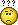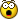# Import and Split CSV - 2

V

I have a slightly different problem to the one posted in "Import and
Split CSV"
my file is small my data in the CSV file looks like this (all on one
sheet):

(2006-04-22 13-00) Sydney Cup Day SEVEN
NAME1
xxxx
xxxx
xxxx
Total for NAME1

NAME2
xxxx
xxxx
xxxx
Total for NAME2

What I would like is when the file is imported for the macro to look
through the CSV selects all data relating to NAME1 (so it selects Name1
and everything below NAME1 till the blank line) copy the data (entire
row) and paste it into a new sheet titled "Name1" or what ever is in
the cell where name1 is. Then repeat this for all subsequent data in
the csv file so you get a sheet titled Name2, Name3...etc with just the
data relating to that name in the sheet.

Is this possible? If not can someone suggest how I would do this? Your
suggestions dont really need to be import based a macro that just
splits up the main data sheet will work just as well. If you dont have
a solution I would appreciate some tips or any advise you have to
offer. Ive been working on this for some time now and i have limited
VBA knowledge but am learning slowly so any help is greatly
appreciared.

Thank you.

B

#### bgeier

You could import the data with either line input or input # to read the
data assign it to variables then do whatever you want with it. It can
be tricky sometimes, but is usually easier than using Excel's import.

V

Sorry bgeier but I have no idea what that means. actuall I do understan
what you saying but what i mean is I have no idea how to do itT

#### Tom Ogilvy

Sub WriteFile()
Dim ff As Long, j As Long
Dim v As Variant
ff = FreeFile()
ReDim v(1 To 10)
Open "C:\Data\TestABC.Txt" For Input As #ff
Line Input #ff, l
j = 0
Do While Not EOF(ff)
j = j + 1
Line Input #ff, l
If Len(Trim(l)) < 1 Then
bk.Worksheets.Count)
ActiveSheet.Name = v(1)
ActiveSheet.Range("A1:J1").Value = v
ReDim v(1 To 10)
j = 0
Else
v(j) = l
End If
Loop
If Len(Trim(v(1))) > 0 Then
bk.Worksheets.Count)
ActiveSheet.Name = v(1)
ActiveSheet.Range("A1:J1").Value = v
End Sub

End Sub

V

Thanks you've helped me before havent you, I remember the name thanks a
lot.

I used the code that you provided below but it says "Compile error
bolck if without end if" how do i correct this this?

Code:
--------------------
Sub WriteFile()
Dim ff As Long, j As Long
Dim v As Variant
ff = FreeFile()
ReDim v(1 To 10)
Open "C:\Test.Txt" For Input As #ff
Line Input #ff, l
j = 0
Do While Not EOF(ff)
j = j + 1
Line Input #ff, l
If Len(Trim(l)) < 1 Then
bk.Worksheets.Count)
ActiveSheet.Name = v(1)
ActiveSheet.Range("A1:J1").Value = v
ReDim v(1 To 10)
j = 0
Else
v(j) = l
End If
Loop
If Len(Trim(v(1))) > 0 Then
bk.Worksheets.Count)
ActiveSheet.Name = v(1)
ActiveSheet.Range("A1:J1").Value = v
End Sub

T

#### Tom Ogilvy

Sorry - after successfully testing it, I added a couple of lines of code to
of those lines. Here is a revision - this has been tested and works fine.

Sub WriteFile()
Dim ff As Long, j As Long
Dim v As Variant
ff = FreeFile()
ReDim v(1 To 10)
Open "C:\Data\TestABC.Txt" For Input As #ff
Line Input #ff, l
j = 0
Do While Not EOF(ff)
j = j + 1
Line Input #ff, l
If Len(Trim(l)) < 1 Then
bk.Worksheets.Count)
ActiveSheet.Name = v(1)
ActiveSheet.Range("A1:J1").Value = v
ReDim v(1 To 10)
j = 0
Else
v(j) = l
End If
Loop
If Len(Trim(v(1))) > 0 Then
bk.Worksheets.Count)
ActiveSheet.Name = v(1)
ActiveSheet.Range("A1:J1").Value = v
End If

End Sub

V

G

#### Guest

After seeing your later post, what I offered doesn't match what I understood
you to describe - so I guess you now want to import it into a worksheet and
then break it up.

B

#### bgeier

Can you attach a coppy of the data to be imported?
That way I will be able to create a macro to import the data and forma
it the way you want.
Or if not, can you send a sample of the file you want changed

T

#### Tom Ogilvy

He already did - find his later post.

B

#### bgeier

My bad, I read the initial post as being how the data is arranged after
it is imported!!

I guess I get the ID 10 T award for today!